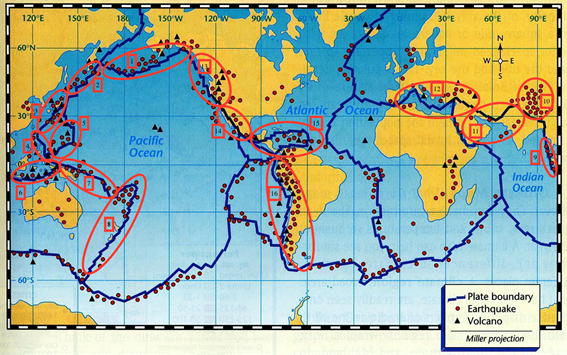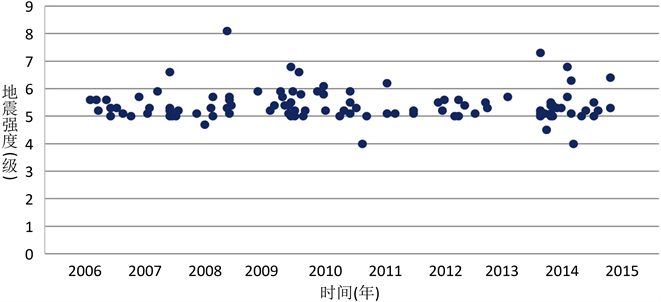# 基于神经网络和灰度预测方法的地震预测模型Earthquake Prediction Model Based on Neural Network and Gray Prediction Theory

DOI: 10.12677/AAM.2018.73031, PDF, HTML, XML, 下载: 948  浏览: 1,813  国家自然科学基金支持

Abstract: Earthquake is a powerful natural disaster. The occurrence of earthquake has brought great disaster to human society. It threatens both the peaceful life and the development of civilization of human being. Therefore, it is necessary to predict the earthquake accurately. In this paper, a long-term prediction model of earthquake was established based on neural network and a short-term prediction model of earthquake was established based on gray prediction theory. The models are used to predict the occurrence of earthquakes in order to reduce the earthquake disaster loss. Firstly, according to the distribution of global pleistoseismic zone, the last 50 years’ seismic data has been preliminarily screened. In this paper, neural network was used to establish long-term earthquake prediction model due to the randomness of seismic activity. It was proved by analyzing the number of times and magnitude of earthquake activity in China that the predicted model was exact. Further, this model was applied to predict the occurrence of earthquake in China in 2018. Then, we analyzed the time and the magnitude of earthquake in the last ten years. Based on gray prediction theory, we established short-term prediction model of earthquake. The accuracy of model was verified by analyzing former data. The gray prediction model can fit the magnitude of the earthquake well.

1. 引言

2. 地震长期预测神经网络模型

2.1. 模型的假设Figure 1. Global pleistoseismic zone distribution map

1) 不考虑远离16个强震活动区的区域所发生的强震对其后一年我国强震发生的影响；

2) 在短期时间(15天)内，各地震带不发生两次或以上强震(发生强震需长时间积蓄大量能量)；

3) 将两个月定为统计强地震的单位时间；

4) 在考虑强震影响时，忽略6.0级以下地震的影响；

5) 假设一段时期内，弱震和中强震的产生有一定的规律，而强震的产生在短期时间内无规律。

2.2. 符号说明

Ms：表示地震等级；

${\omega }_{ij}$ ：表示第i年j区的最大震级强度；

${m}_{ij}$ ：归一化处理后第i年j区的震级指数。

2.3. 神经网络模型的建立与求解

BP神经网络(Back Propagation Network)是一种迭代梯度算法，用于求解前馈网络的实际输出与期望输出间的最小均方值  。BP神经网络的分类功能来自神经元节点的非线性，所以，它可以实现由N维空间向M维空间的高度非线性映射，它具有较强的自适应性和自学习功能，而且具有高度的容错性。当个别输入信号值出现畸变或干扰时一般都不会影响识别结果。因此从理论上讲，利用神经网络模型，可以更好地进行地震预测  。

${m}_{ij}=\frac{{\omega }_{ij}-\underset{j}{\mathrm{min}}\left({\omega }_{ij}\right)}{\underset{j}{\mathrm{max}}\left({\omega }_{ij}\right)-\underset{j}{\mathrm{min}}\left({\omega }_{ij}\right)}$

BP神经网络的输入节点，输出节点为下一个年的最大地震震级。根据各年中各强震活动区以及中国的最大震级统计数据，可以通过选取近40年的34个样本，以15年以及其后一年的数据作为训练集，对神经网络模型进行训练，通过选择不同的函数进行拟合，得出神经网络模型。将剩下的样本作为测试集合，通过测试验证，由模型得出的预测值与真实值之间的误差平均为0.5级。由模型得出的预测值与真实值之间的误差统计如表1所示。

2.4. 模型的修正

$准确率=\frac{准确预测样本数}{样本总数}$Table 1. Error statistical table of the predicted value of neural network model and the real valueTable 2. The predicted value of neural network model and the real value

3. 地震短期预测灰度模型

3.1. 短期预测的理论依据

3.2. 灰度预测模型的建立与求解

$y=\mathrm{sin}\left(\frac{1}{\alpha }x+\beta \right)+\epsilon$Figure 2. The time and magnitude of earthquake in China from 2006 to 2015

$y=\mathrm{sin}\left(\frac{1}{40}x+\frac{\pi }{24}+5.3\right)$

2008年是中国地震多发年，为验证模型效果，以2008至2009上半年为例，模型结果与真实值对比统计如表3所示。

4. 地震预测模型评价与讨论

4.1. 模型的优点

4.2. 模型的缺点Table 3. The real value of earthquake magnitude and fitted value of gray prediction model

5. 结语

  陈运泰. 地震预测: 回顾与展望[J]. 中国科学(D辑: 地球科学), 2009, 39(12): 1633-1658.  张培震, 邓起东, 张竹琪, 李海兵. 中国大陆的活动断裂、地震灾害及其动力过程[J]. 中国科学: 地球科学, 2013, 43(10): 1607-1620.  吴忠良, 陈运泰. 地震预测与统计物理[J]. 物理, 2002, 31(6): 365-371.  丁国瑜. 中国岩石圈动力学概论[M]. 北京: 地震出版社, 1991.  朝娣, 吴小平, 雷兴林, 冒蔚, 孙楠. 长周期潮汐与全球地震能量释放[J]. 地球物理学报, 2013, 56(10): 3425-3433.  赵利飞, 王炜. 利用神经网络技术对华东地区进行地震预测[J]. 地震学刊, 2002, 22(4): 21-25.  付杰. 基于神经网络的短期地震预测模型及其应用[D]: [硕士学位论文]. 南京: 东南大学, 2016.  魏红梅, 黄世源, 贺曼秋. 基于MATLAB工具箱的地震预测模型[J]. 东北地震研究, 2007, 23(3): 64-68.  谢家智, 车四方, 林涌. 基于随机权神经网络的地震灾害经济损失评估与预测[J]. 灾害学, 2017, 32(1): 1-4 + 10.  周硕愚, 吴云, 江在森. 地震大地测量学及其对地震预测的促进——50年进展、问题与创新驱动[J]. 大地测量与地球动力学, 2017, 37(6): 551-562.  张培震, 等. 天山活动构造[M]. 北京: 地震出版社, 2000.  张晓东, 蒋海昆, 黎明晓. 地震预测与预警探讨[J]. 中国地震, 2008, 24(1): 67-76.  马钦忠. 中外几次重要地震预测与预报结果之启示[J]. 地震学报, 2014, 36(3): 500-513.